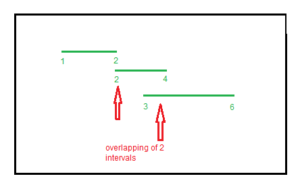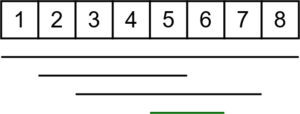GeeksforGeeks App
Open AppBrowser
Continue

# Maximum number of overlapping Intervals

Given different intervals, the task is to print the maximum number of overlap among these intervals at any time.

Examples:

Input: v = {{1, 2}, {2, 4}, {3, 6}}
Output:
The maximum overlapping is 2(between (1 2) and (2 4) or between (2 4) and (3 6))Input: v = {{1, 8}, {2, 5}, {5, 6}, {3, 7}}
Output:
The maximum overlapping is 4 (between (1, 8), (2, 5), (5, 6) and (3, 7))Approach:

• The idea is to store coordinates in a new vector of pair mapped with characters ‘x’ and ‘y’, to identify coordinates.
• Sort the vector.
• Traverse the vector, if an x coordinate is encountered it means a new range is added, so update count and if y coordinate is encountered that means a range is subtracted.
• Update the value of count for every new coordinate and take maximum.

Below is the implementation of the above approach:

## C++

 `// C++ program that print maximum``// number of overlap``// among given ranges``#include ``using` `namespace` `std;` `// Function that print maximum``// overlap among ranges``void` `overlap(vector > v)``{``    ``// variable to store the maximum``    ``// count``    ``int` `ans = 0;``    ``int` `count = 0;``    ``vector > data;` `    ``// storing the x and y``    ``// coordinates in data vector``    ``for` `(``int` `i = 0; i < v.size(); i++) {` `        ``// pushing the x coordinate``        ``data.push_back({ v[i].first, ``'x'` `});` `        ``// pushing the y coordinate``        ``data.push_back({ v[i].second, ``'y'` `});``    ``}` `    ``// sorting of ranges``    ``sort(data.begin(), data.end());` `    ``// Traverse the data vector to``    ``// count number of overlaps``    ``for` `(``int` `i = 0; i < data.size(); i++) {` `        ``// if x occur it means a new range``        ``// is added so we increase count``        ``if` `(data[i].second == ``'x'``)``            ``count++;` `        ``// if y occur it means a range``        ``// is ended so we decrease count``        ``if` `(data[i].second == ``'y'``)``            ``count--;` `        ``// updating the value of ans``        ``// after every traversal``        ``ans = max(ans, count);``    ``}` `    ``// printing the maximum value``    ``cout << ans << endl;``}` `// Driver code``int` `main()``{``    ``vector > v``        ``= { { 1, 2 }, { 2, 4 }, { 3, 6 } };``    ``overlap(v);``    ``return` `0;``}`

## Java

 `// Java program that print maximum``// number of overlap among given ranges``import` `java.util.*;``import` `java.lang.*;``import` `java.io.*;``class` `GFG{``    ` `static` `class` `pair``{``    ``int` `first;``    ``char` `second;``    ` `    ``pair(``int` `first, ``char` `second)``    ``{``        ``this``.first = first;``        ``this``.second = second;``    ``}``}` `// Function that print maximum``// overlap among ranges``static` `void` `overlap(``int``[][] v)``{``    ` `    ``// Variable to store the maximum``    ``// count``    ``int` `ans = ``0``;``    ``int` `count = ``0``;``    ``ArrayList data = ``new` `ArrayList<>();``    ` `    ``// Storing the x and y``    ``// coordinates in data vector``    ``for``(``int` `i = ``0``; i < v.length; i++)``    ``{``        ` `        ``// Pushing the x coordinate``        ``data.add(``new` `pair(v[i][``0``], ``'x'``));``  ` `        ``// pushing the y coordinate``        ``data.add(``new` `pair(v[i][``1``], ``'y'``));``    ``}``    ` `    ``// Sorting of ranges``    ``Collections.sort(data, (a, b) -> a.first - b.first);``  ` `    ``// Traverse the data vector to``    ``// count number of overlaps``    ``for``(``int` `i = ``0``; i < data.size(); i++)``    ``{``        ` `        ``// If x occur it means a new range``        ``// is added so we increase count``        ``if` `(data.get(i).second == ``'x'``)``            ``count++;``  ` `        ``// If y occur it means a range``        ``// is ended so we decrease count``        ``if` `(data.get(i).second == ``'y'``)``            ``count--;``  ` `        ``// Updating the value of ans``        ``// after every traversal``        ``ans = Math.max(ans, count);``    ``}``  ` `    ``// Printing the maximum value``    ``System.out.println(ans);``}` `// Driver code``public` `static` `void` `main(String[] args)``{``    ``int``[][] v = { { ``1``, ``2` `},``                  ``{ ``2``, ``4` `},``                  ``{ ``3``, ``6` `} };``    ``overlap(v);``}``}` `// This code is contributed by offbeat`

## Python3

 `# Python3 program that print maximum``# number of overlap``# among given ranges` `# Function that print maximum``# overlap among ranges``def` `overlap(v):` `    ``# variable to store the maximum``    ``# count``    ``ans ``=` `0``    ``count ``=` `0``    ``data ``=` `[]` `    ``# storing the x and y``    ``# coordinates in data vector``    ``for` `i ``in` `range``(``len``(v)):` `        ``# pushing the x coordinate``        ``data.append([v[i][``0``], ``'x'``])` `        ``# pushing the y coordinate``        ``data.append([v[i][``1``], ``'y'``])` `    ``# sorting of ranges``    ``data ``=` `sorted``(data)` `    ``# Traverse the data vector to``    ``# count number of overlaps``    ``for` `i ``in` `range``(``len``(data)):` `        ``# if x occur it means a new range``        ``# is added so we increase count``        ``if` `(data[i][``1``] ``=``=` `'x'``):``            ``count ``+``=` `1` `        ``# if y occur it means a range``        ``# is ended so we decrease count``        ``if` `(data[i][``1``] ``=``=` `'y'``):``            ``count ``-``=` `1` `        ``# updating the value of ans``        ``# after every traversal``        ``ans ``=` `max``(ans, count)` `    ``# printing the maximum value``    ``print``(ans)` `# Driver code``v ``=` `[ [ ``1``, ``2` `], [ ``2``, ``4` `], [ ``3``, ``6` `] ]``overlap(v)` `# This code is contributed by mohit kumar 29`

## C#

 `// Include namespace system``using` `System;``using` `System.Collections.Generic;` `public` `class` `GFG``{``     ``class` `pair``    ``{``        ``public` `int` `first;``        ``public` `char` `second;``        ``public` `pair(``int` `first, ``char` `second)``        ``{``            ``this``.first = first;``            ``this``.second = second;``        ``}``    ``}``  ` `    ``// Function that print maximum``    ``// overlap among ranges``    ``public` `static` `void` `overlap(``int``[,] v)``    ``{``      ` `        ``// Variable to store the maximum``        ``// count``        ``var` `ans = 0;``        ``var` `count = 0;``        ``var` `data = ``new` `List();``      ` `        ``// Storing the x and y``        ``// coordinates in data vector``        ``for` `(``int` `i = 0; i < v.GetLength(0); i++)``        ``{``          ` `            ``// Pushing the x coordinate``            ``data.Add(``new` `pair(v[i,0], ``'x'``));``          ` `            ``// pushing the y coordinate``            ``data.Add(``new` `pair(v[i,1], ``'y'``));``        ``}``      ` `        ``// Sorting of ranges``        ``data.Sort((a,b)=>a.first - b.first);``      ` `        ``// Traverse the data vector to``        ``// count number of overlaps``        ``for` `(``int` `i = 0; i < data.Count; i++)``        ``{``          ` `            ``// If x occur it means a new range``            ``// is added so we increase count``            ``if` `(data[i].second == ``'x'``)``            ``{``                ``count++;``            ``}``          ` `            ``// If y occur it means a range``            ``// is ended so we decrease count``            ``if` `(data[i].second == ``'y'``)``            ``{``                ``count--;``            ``}``          ` `            ``// Updating the value of ans``            ``// after every traversal``            ``ans = Math.Max(ans,count);``        ``}``      ` `        ``// Printing the maximum value``        ``Console.WriteLine(ans);``    ``}``  ` `    ``// Driver code``    ``public` `static` `void` `Main(String[] args)``    ``{``        ``int``[,] v = {{1, 2}, {2, 4}, {3, 6}};``        ``GFG.overlap(v);``    ``}``}` `// This code is contributed by aadityaburujwale.`

## Javascript

 ``

Output:

`2`

Time Complexity: O(N log N), for sorting the data vector.
Auxiliary Space: O(N), for creating an additional array of size N.

My Personal Notes arrow_drop_up